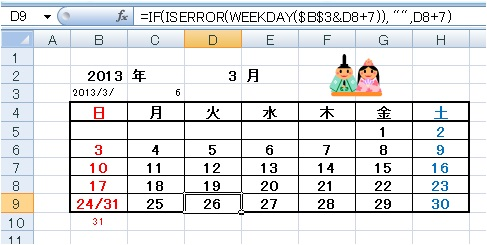Japanese

## Calendar using Excel

Creating calendar is proposed here though there may exist a more simple method.

Figure 1．A calendar automatically appears if you enter year and month. As the cells b3, c3, b10, and c10 are visually obstructive; alteration of font color of these cells to white may be the simple method.

(note) 『 =weekday("2013/3/1") 』returns a number of week of the specified date (March 1, 2013) …（this date is Friday i.e. 6 days count from Sunday, therefore the WEEKDAY-function returns 6 ）

『 =weekday("2010/2/29") 』 returns #VALUE! error because of absence of such date, but 『 =iserror(weekday("2010/2/29")) 』 gives TRUE.

You can get a sample of above calendar if you copy the subsequent data to your Excel Sheet. The string "sample" must be set in A1 cell.

 sample 2013 year 3 month =B2&"/"&D2&"/" =WEEKDAY(B3&"1") Sun Mon Tue Wed Thu Fri Sat =IF(C3=1,1,"") =IF(B5="",IF(\$C\$3=2,1,""),B5+1) =IF(C5="",IF(\$C\$3=3,1,""),C5+1) =IF(D5="",IF(\$C\$3=4,1,""),D5+1) =IF(E5="",IF(\$C\$3=5,1,""),E5+1) =IF(F5="",IF(\$C\$3=6,1,""),F5+1) =IF(G5="",1,G5+1) =H5+1 =B6+1 =C6+1 =D6+1 =E6+1 =F6+1 =G6+1 =B6+7 =C6+7 =D6+7 =E6+7 =F6+7 =G6+7 =H6+7 =B7+7 =C7+7 =D7+7 =E7+7 =F7+7 =G7+7 =H7+7 =IF(ISERROR(WEEKDAY(\$B\$3&B8+7)), "",B8+7&IF(B10="","","/"&B10)) =IF(ISERROR(WEEKDAY(\$B\$3&C8+7)), "",C8+7&IF(C10="","","/"&C10)) =IF(ISERROR(WEEKDAY(\$B\$3&D8+7)), "",D8+7) =IF(ISERROR(WEEKDAY(\$B\$3&E8+7)), "",E8+7) =IF(ISERROR(WEEKDAY(\$B\$3&F8+7)), "",F8+7) =IF(ISERROR(WEEKDAY(\$B\$3&G8+7)), "",G8+7) =IF(ISERROR(WEEKDAY(\$B\$3&H8+7)), "",H8+7) =IF(ISERROR(WEEKDAY(\$B\$3&B8+14)), "",B8+14) =IF(ISERROR(WEEKDAY(\$B\$3&C8+14)), "",C8+14)